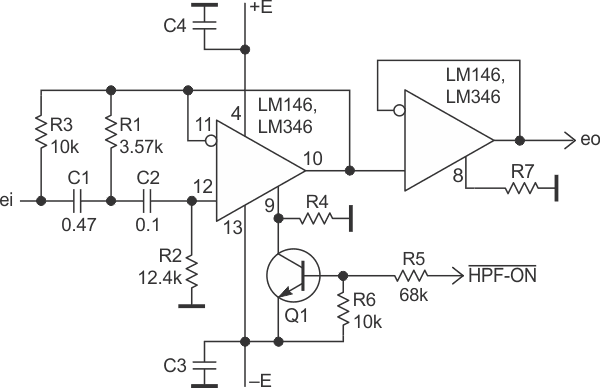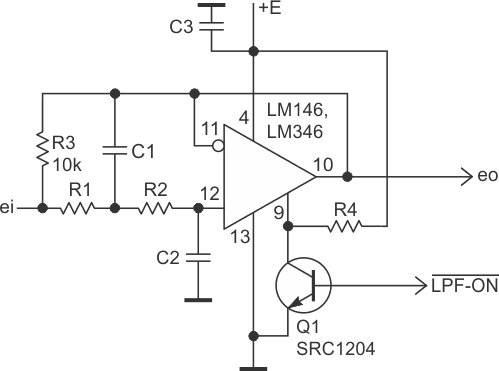# Simple switchable filter

## Texas Instruments LM146 LM346 LM4250

Figure 1 shows how a Sallen-Key high pass filter (HPF), can be switched on/off using only one extra transistor and resistor.Figure 1. A Sallen-Key HPF filter topology that can be switched on/off using an extra transistor and resistor.

This solution seems adequate for the Sallen-Key filter since the topology is rather simple itself.

The op amp here is a programmable op amp with a sinking setting current ISET (i.e., the current through the setting resistor R4 that sinks into op amp). The LM146 and LM346 are the op amps of this kind.

An op amp which uses sourcing setting current (like LM4250) can also be used with a simple mirrored modification of this circuit. For example, Q1 becomes a PNP type transistor and should be connected instead to the +E terminal.

Resistor R3 represents a roundabout way when the op amp is switched off. When the transistor Q1 is off, the setting current flows into the ISET terminal (pin 9). So, the op amp is on and has a very low output impedance that comprises of the resistor R3 in a voltage divider which effectively eliminates the roundabout way (more than 80 dB), leaving the filtered output.Figure 2. A LPF topology that can be switched on/off by using unipolar power and a transistor Q1 (SRC1204) with built-in bias resistors.

If the transistor Q1 is on, the current ISET flows into Q1 and cannot activate the ISET terminal. This is because of the threshold voltage (0.8 V to 0.9 V) of the terminal ISET. So, the op amp is off, and its output is effectively disconnected from the circuit.

Now the output is determined by a voltage divider composed of the resistor R3 and the impedance of the load. To emphasize this, the next cascade is shown as a buffer, which is not obligatory. Note: the components of the filter (R1 and C1) are connected in parallel to the resistor R3, this cannot be neglected.

A version of an LPF is shown in Figure 2. It uses unipolar power and a transistor Q1 (SRC1204) with built-in bias resistors which makes the circuit even simpler.

EDN